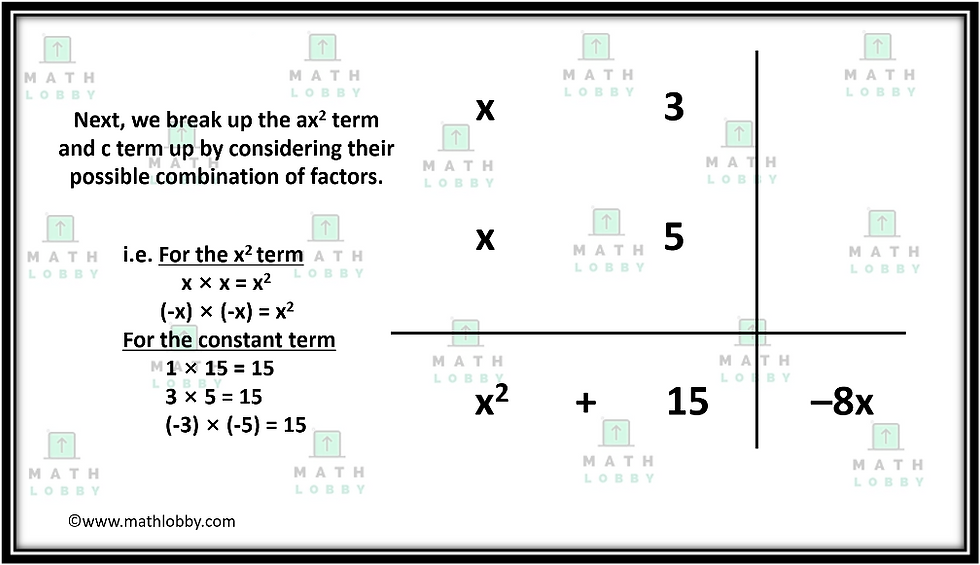top of page
Search

# How to factorise quadratic equations using multiplication frame

Updated: Sep 10, 2021In this note, you will learn:

· How to factorize quadratic equations of the form x^2 + bx + c, where c > 0 ( and b < 0)?

Before we begin, I would like to say that factorization of quadratic equations is actually very systematic and easy! As long as you follow the steps, I am sure that you will have no issues with mastering it at the back of your hands. Without further ado, let’s begin!

## How to factorize quadratic equations of the form x^2 + bx + c, where c > 0 (and b < 0)?

Factorization of a quadratic equation of the form x^2 + bx + c, where c > 0 and b < 0 is actually not much different from the factorization of a quadratic equation with c > 0 and b > 0.

The only difference is that you will have to consider the both the positive and negative factors of the constant term when doing the multiplication. All of this will be clearer when you see the example which I am about to show you.

For this example, let’s take x^2 – 8x + 15 as the problem we are trying to solve here.As shown in the diagram above, the first step is to place the ax^2 term and the c term on the left side of the multiplication frame, followed by the bx term on the right side of the multiplication frame.

Do note that whenever you are placing terms into the multiplication frame, you MUST remember to bring along its OPERATION SIGN with it. It is very important as with and without the signs can yield very different answers!Next, we will have to break up the ax^2 term and c term by considering their possible combination of factors. As shown above in the diagram, there are multiple possible combination of factors to consider. Hence, we can use the guess and check method.

The guess and check method basically means that we can try the possible pairs of combination until we yield the correct answer. However, to try every possible pair of combinations can be very tedious and time-consuming so that is not what you will want to do in an examination setting. Hence, we can use a little bit of logic and reasoning to, at the very least, narrow down the options we have to consider before trying.With that being said, we can see that for the bx term, there is a negative sign that comes along with it. Hence, it would be wise to use the negative factors of 15: -3 and -5, since the sum adds up to -8 and (-3) ×(-5) = 15.

And there you have it! The answer after factorizing x^2 – 8x + 15 is equals to (x – 3) (x – 5)!

And that’s all for today, students! Math Lobby hopes that after this article, you have a clear understanding on the methods of how to factorize quadratic equations using the multiplication frame!

If you have any pending questions, please do go on to our Facebook page, Instagram or contact us directly at Math Lobby! We have certified mathematics tutors to aid you in your journey to becoming a better student!

As always: Work hard, stay motivated and we wish all students a successful and enjoyable journey with Math Lobby!

Visit and FOLLOW our Instagram page at https://www.instagram.com/mathlobbymotivation/

*

*

*